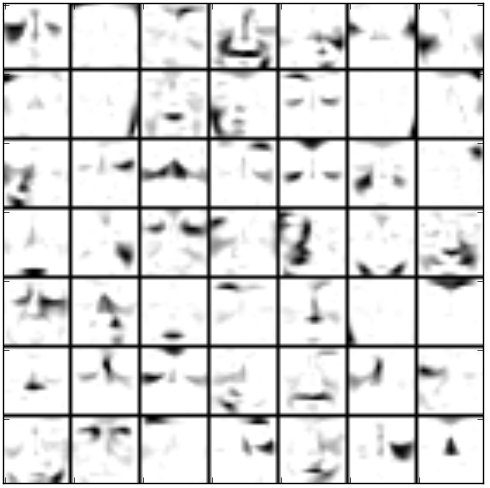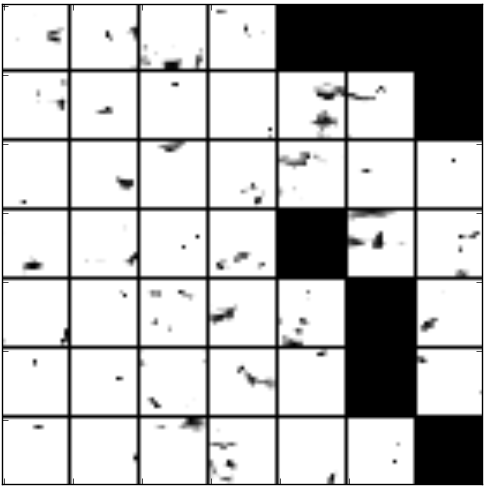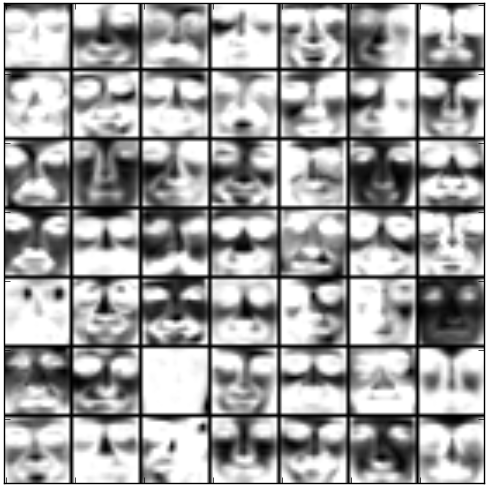# CBCL Images (examples.cbcl_images)¶

In this example of image processing we consider the problem demonstrated in [Lee1999].

We used the CBCL face images database consisting of 2429 face images of size 19 x 19. The facial images consist of frontal views hand aligned in a 19 x 19 grid. Each face image is preprocessed. For each image, the greyscale intensities are first linearly scaled, so that the pixel mean and standard deviation are equal to 0.25, and then clipped to the range [0, 1].

Note

The CBCL face images database used in this example is not included in the datasets. If you wish to perform the CBCL data experiments, start by downloading the images. Download links are listed in the datasets. To run the example, uncompress the data and put it into corresponding data directory, namely the extracted CBCL data set must exist in the CBCL_faces directory under datasets. Once you have the data installed, you are ready to start running the experiments.

We experimented with the following factorization algorithms to learn the basis images from the CBCL database: Standard NMF - Euclidean, LSNMF, SNMF/R and SNMF/L. The number of bases is 49. Random Vcol algorithm is used for factorization initialization. The algorithms mostly converge after less than 50 iterations.

Unlike vector quantization and principal components analysis ([Lee1999]), these algorithms learn a parts-based representations of faces and some also spatially localized representations depending on different types of constraints on basis and mixture matrix. Following are 7 x 7 montages of learned basis images by different factorization algorithms.Basis images of LSNMF obtained after 50 iterations on original CBCL face images. The bases trained by LSNMF are additive but not spatially localized for representation of faces. 10 subiterations and 10 inner subiterations are performed (these are LSNMF specific parameters).Basis images of NMF obtained after 50 iterations on original CBCL face images. The images show that the bases trained by NMF are additive but not spatially localized for representation of faces.Basis images of SNMF/L obtained after 10 iterations on original CBCL face images. The bases trained from LSNMF/L are both additive and spatially localized for representing faces. LSNMF/L imposes sparseness constraints on basis matrix, whereas LSNMF/R imposes sparseness on mixture matrix. Therefore obtained basis images are very sparse as it can be shown in the figure. The Euclidean distance of SNMF/L estimate from target matrix is 1827.66.Basis images of SNMF/R obtained after 10 iterations on original CBCL face images. The images show that the bases trained by NMF are additive but not spatially localized for representation of faces. The Euclidean distance of SNMF/R estimate from target matrix is 3948.149.

To run the example simply type:

```python cbcl_images.py
```

or call the module’s function:

```import nimfa.examples
nimfa.examples.cbcl_images.run()
```

Note

This example uses matplotlib library for producing visual interpretation of basis vectors. It uses PIL library for displaying face images.

nimfa.examples.cbcl_images.factorize(V)

Perform LSNMF factorization on the CBCL faces data matrix.

Return basis and mixture matrices of the fitted factorization model.

Parameters: V (numpy.matrix) – The CBCL faces data matrix.
nimfa.examples.cbcl_images.plot(W)

Plot basis vectors.

Parameters: W (numpy.matrix) – Basis matrix of the fitted factorization model.
nimfa.examples.cbcl_images.preprocess(V)

Preprocess CBCL faces data matrix as Lee and Seung.

Return normalized and preprocessed data matrix.

Parameters: V (numpy.matrix) – The CBCL faces data matrix.

Read face image data from the CBCL database. The matrix’s shape is 361 (pixels) x 2429 (faces).

Step through each subject and each image. Images’ sizes are not reduced.

Return the CBCL faces data matrix.

nimfa.examples.cbcl_images.run()

Run LSNMF on CBCL faces data set.

# Nimfa